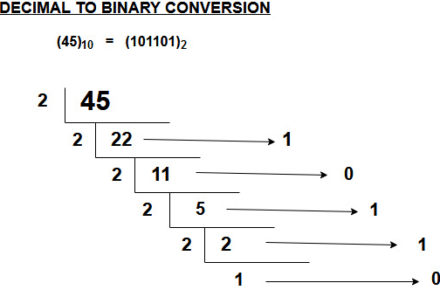# How to Prepare for Computer Aptitude and Questions for Practice – PART 1

Dear Banking Aspirant,

IBPS PO 2017 Mains Examination is happening on the 26th November 2017 and we hope you are preparing in full swing to ace the exam with your exam strategy and other things. We were getting many requests from the aspirants for the Computer Aptitude questions which are asked along with Reasoning Ability in the Mains Examination of the IBPS PO 2017. So here we are to explain the tips and tricks to crack the Computer Aptitude section in the IBPS PO 2017.

The Numerical Ability will be the hard one and usually in the mains examination computer awareness questions will be asked but nowadays apart from some exams, SBI and IBPS (not for RRB) have been following this strategy to include Computer section in the reasoning ability to increase the level of difficulty. There are many patterns asked and many possibilities are there. There aren’t much exams which had computer aptitude , so as of now the patterns which are known to the aspirants are less in number, so we will update this with further addition to these in the future.

The Questions were asked either in the Puzzle Form or in Flowchart and Diagramatic method. So we will discuss the Puzzle form and coded ones in this post and the rest we will discuss in detail in another post. Conversion from one form to another forms the majority in the computer aptitude, so let’s also discuss that in detail.

Check the Pattern of the IBPS PO 2017 Mains Examination and also know the different topics which are asked in the yesteryear exams.

Conversion of Numbers

There are four types of number forms and they are binary, decimal, octal and hexadecimal. The conversion mainly happens within this four forms and mostly there are two methods in this which can solve all the conversions. but before that  you need to know the numbers and the base involved in the numbering system of each one.

Binary

Numbers Used: 0 & 1

Base : 2

The Rightmost number is called as the Most Significant Bit (MSB)  and the leftmost number in it is called as the Least Significant Bit (LSB)

Decimal

Numbers Used: 0, 1,2,3,4,5,6,7,8,9

Base : 10

Octal

Numbers Used: 0,1,2,3,4,5,6,7

Base : 8

Numbers Used: 0,1,2,3,4,5,6,7,8,9,10(A),11(B), 12(C), 13(D), 14(E), 15(F)

Base : 16

So the first one in the conversion will be from DECIMAL to other things because it will be easier and all one needs to know is subsequent division (refer the diagram for info). So you can follow the same conversion process but with number eight for Decimal to Octal and with number sixteen if you want to convert from decimal to hexadecimal.

DECIMAL TO BINARY

Steps :

1. Divide the Given number by 2
2. For the next iteration , get the quotient
3. Get the remainder which is used to determine the binary number
4. Repeat the steps until the iteration gets a 1 as quotient.OTHER BASE SYSTEM TO DECIMAL NUMBER SYSTEM

Steps

• Determine the  value of each digit (Refer the Diagram)
• Multiply the obtained column values by the digits in the corresponding columns.
• Sum the products obtained
• The total is the equivalent value in decimal. (Same can be done with octal and hexadecimal, just replace the base number of each one for the conversion)Conversion of Hexadecimal & Octal to Binary

Steps

• Take the individual octal number and convert it into pair of three binary numbers
• Group all the binary numbers into a single binary number

E.g:- 17 to binary which is in the octal form.

Form a three pair number for 1 and 7 individually

1 – 001 & 7 – 111 respectively.

Together they form a compete binary number as (001111)

Conversion of Binary to Hexadecimal and Octal

Steps

• Group the Binary Digits into a 3 set pair for octal and 4 set pair for the Hexadecimal number.
• Convert the Pair into its equivalent Octal or Hexadecimal Number

• Take into a 4 set pair , so the number goes like this 0001 0101 which is a two, four set pair.
• Convert the above four set pair into the equivalent hexadecimal number which is 1516.
• So ( 0001 will be the hexadecimal equivalent of 1 and 0101 is the hexadecimal equivalent of 5, so both combined to form 15)

NOTE : Same thing goes for OCTAL conversion but , you have to take it as a set of 3 pairs. (E.g:- (xxx))

Apart from this Descriptive section is also important for the IBPS PO Mains Examination 2017, So Please have a look at the descriptive part section and how to attend them from the links given below.

So we hope these would have enlightened you about the various number system conversion methods. We will be uploading the Questions for the Computer Aptitude in a Separate post after the topics are explained and until then , please book mark this page to know the updated articles in the Computer Aptitude section. All the questions will be given as a PDF for the aspirants, so that you can download it and practice the questions at your own time.